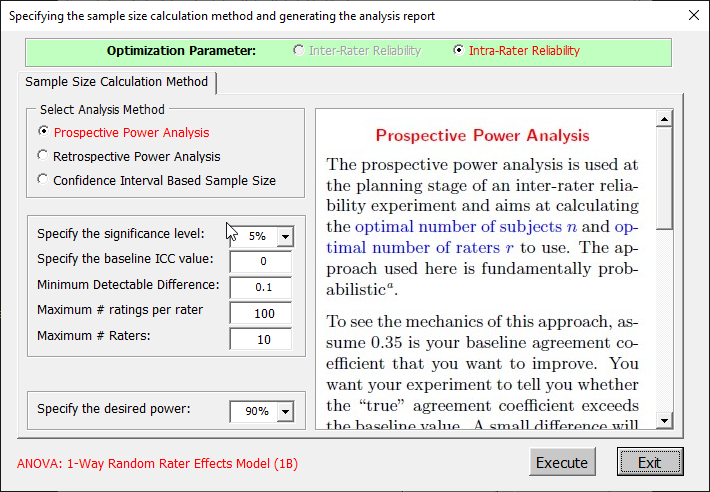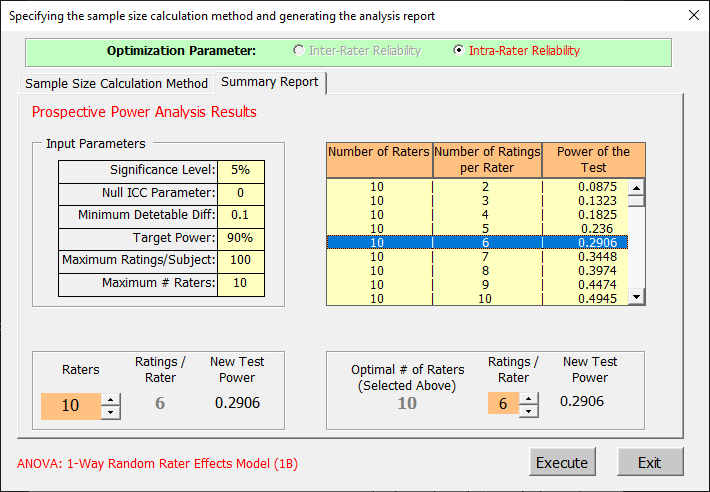﻿ Intraclass Correlation Sample Size Determination Prospective Power Analysis under the one-way random rater effects modelAgreeStat Analytics

## Intraclass Correlation Sample Size DeterminationProspective Power Analysis/1-Way Random Rater Effects Model

Problem

Assume you are in the planning stage of an intra-rater reliability experiment, and that the one-way random rater effects design will be adopted.  However, you do not know how many raters or ratings per rater should be used.

AgreeStat360 can be used to determine the optimal number of raters as well as the optimal number of ratings to take per rater.  The input data needed to run this module is described in the figure below. To allow the software to suggest the most meaningful recommendations, it is essential to provide the following:

• The maximum number of raters and number of ratings per rater you would consider as shown in the figure. In this example I assume no more than 10 raters will participate in the experiment, each of whom will produce no more than 100 ratings.

• The desired statistical power for detecting the Minimum Detectable Diffefference (MDD) of 0.1 would be 90%. Feel free to modify the MDD and desired power values.Analysis with AgreeStat/360

To see how AgreeStat360 processes this dataset to produce various agreement coefficients, please play the video below.  This video can also be watched on youtube.com for more clarity if needed.

Results

The output that AgreeStat360 produces is shown below.  It contains among other things, the 3-column "Power Table" on the right side showing the magnitude of the power associated with the number of raters and number of ratings per rater in the first 2 columns.

• The highlighted row shows that with 10 raters and 6 ratings per rater for a total of 60 ratings, one can expect to achieve a power of 0.0.2906, which is very low.  To obtain a higher power, one may need to increase the MDD value as one possible option.

• If a particular row is of interest due to the number of ratings per rater (e.g. 6), you may then use the bottom left box to modify the number of raters only and observe how that affects the power.

• If a particular row is of interest due to the number of raters (e.g. 10), you may then use the bottom right box to modify the number of ratings per rater only and observe how that affects the power.